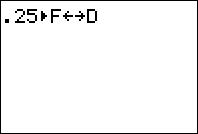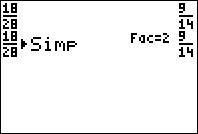# Activities

••• ##### Subject Area

• Math: Middle Grades Math: Algebraic Thinking
• Math: Middle Grades Math: Number Sense

• ##### Author6-8

45 Minutes

• ##### Device
• TI-73 Explorer™
• TI-Navigator™
• ##### Software

LearningCheck™ Creator
TI Connect™

## Different Looks, Same Values#### Activity Overview

In this activity, students explore the meaning and purpose of equivalent fractions. They also practice writing fractions that meet a given criteria.

#### Key Steps

•In this activity, students explore equivalent fractions. They can use the Fraction Decimal conversion and Simplification features on the TI-73 Explorer™ to help convert between decimals and fractions and to rename fractions.

•When using the SIMP key, students will also see the factor by which the fraction was simplified. They will also find factions between two given numbers and write equivalents fractions representing a diagram.